output.to from Sideway
Draft for Information Only

# Moment of a force in 2D about a point

When an application is subjected to coplanar forces, forces in a plane and the effect of the depth on structure analysis is negligible, the problem can be simplified into two dimensional analysis. Because the direction of moment of a force is always normal to the plane joining the reference point and the line of action, the moment of forces in a plane about a point can therefore refer to the sense of the moment about a point.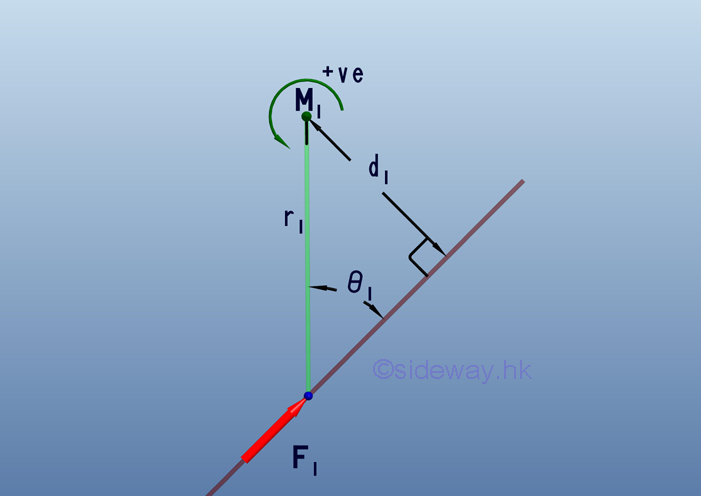According to the right hand rule, a counterclockwise moment about a reference point can be taken as positive, while a clockwise moment about a reference point can be taken as negative.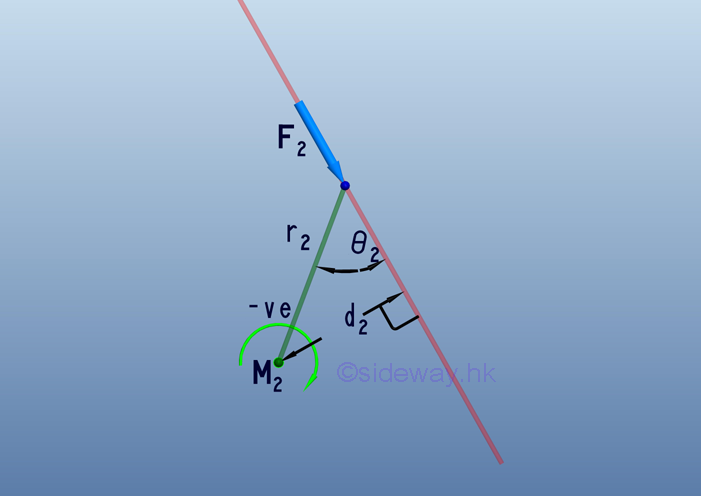The total moment of forces about a reference point can be determined by adding up all moments of forces.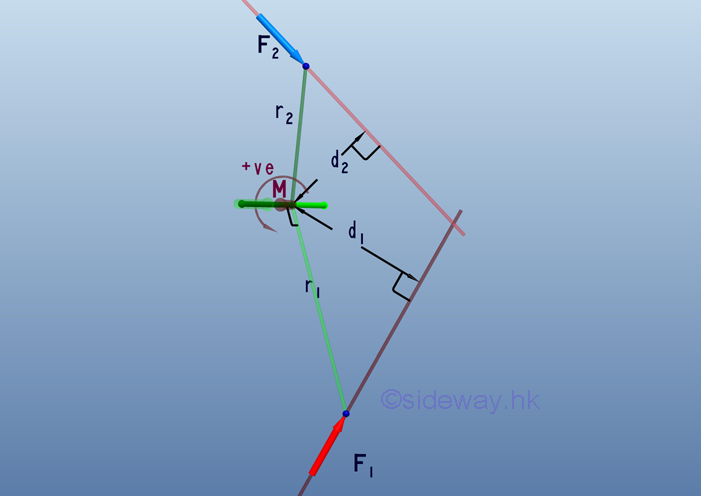Therefore, the magnitude of the effective moment , M, can be expressed as:Moment of Concurrent Forces

For concurrent forces, the moment of the resultant of concurrent forces is also equal to the resultant force times the prependicular distance between the reference point and the line of action of the rusultant force. The effective moment can also be computed by adding up all individual moment of concurrent forces.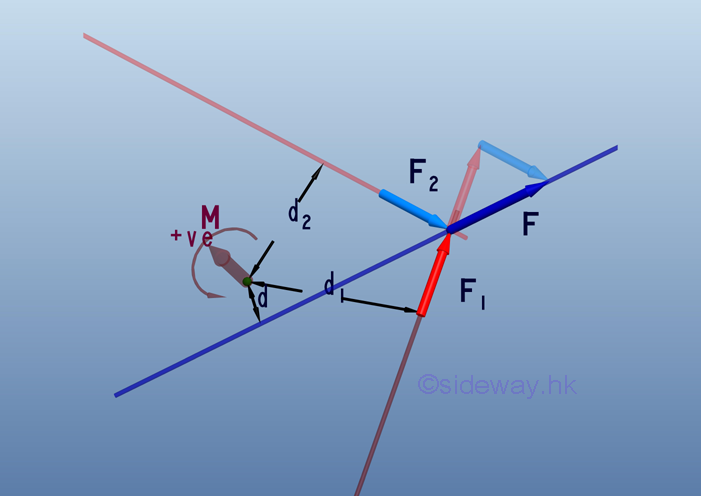Therefore, the magnitude of the moment , M, can be expressed as: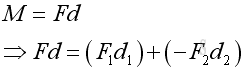Moment Decomposition

Since the effective moment of concurrent forces about a reference point is equal to the sum of the moments of the concurrent forces as stated in Varignon's Theorem. In other words, moment of a force can also be decomposed through force decomposition.

A force, F in a plane can be decomposed into two retangular components, Fx and Fy. The moment, M of force, F can therefore be decomposed into MFx and MFy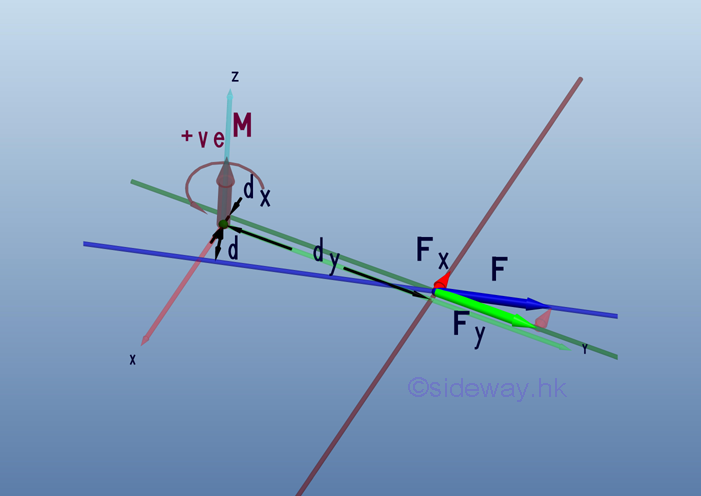Therefore, the magnitude of the moment , M, can be expressed as: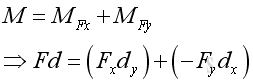Moment Vector

When the force vector is decomposed into two rectangular components, the corresponding perpendicular distance components are also equal to the magnitudes of the two rectangular components of the postition vector, r of the point of application. And the moment vector, M becomes the third dimension of the rectangular coordinate system.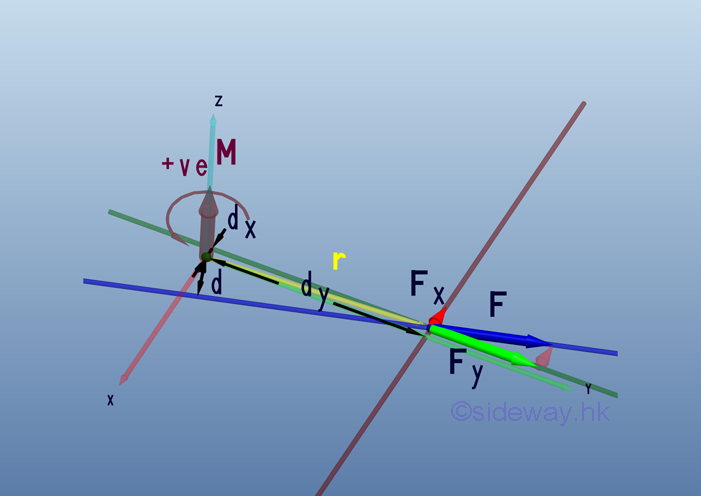Therefore the force vector, F and position vector, r can be expressed as: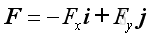andThe moment of a force, M about a reference point can be defined as the vector product of r and F.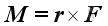ImplySince the magnitude of vector product is the product of the magnitudes of the two vectors and the sine of the angle formed by the vectors, imply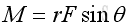Therefore, the vector product of a unit vector with itselt is equal to a zero vector, imply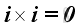andFor the sense of the direction, the vector product of two different unit vectors is equal to the unit vector in the third dimension according to the right hand rule, imply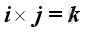and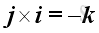Substitute all variables into the moment vector equation, implyID: 110600001 Last Updated: 6/9/2011 Revision: 1 Ref:References

1. I.C. Jong; B.G. rogers, 1991, Engineering Mechanics: Statics and Dynamics
2. F.P. Beer; E.R. Johnston,Jr.; E.R. Eisenberg, 2004, Vector Mechanics for Engineers: StaticsHome 5

Management

HBR 3

Information

Recreation

Culture

Chinese 1097

English 337

Computer

Hardware 154

Software

Application 207

Latex 35

Manim 203

Numeric 19

Programming

Web 285

Unicode 504

HTML 65

CSS 63

SVG 6

ASP.NET 210

OS 422

Python 64

Knowledge

Mathematics

Algebra 84

Geometry 25

Calculus 67

Engineering

Mechanical

Rigid Bodies

Statics 92

Dynamics 37

Control

Natural Sciences

Electric 27